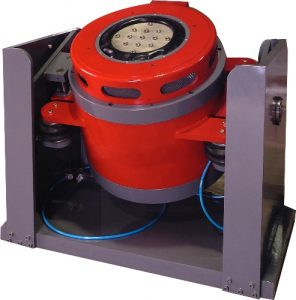## Electrodynamic ShakerThe system rating are prepared as per directions of International Standards Organisation document ISO 5344. Following information assists in selecting a suitable configuration system for your intended application.

To frame out ratings of a vibration system based upon your test application calls for extensive calculations and analysis as all parameters are interlinked to each other very closely with specimen mass, dimension, frequency range and test axes playing a vital role.

The selection of a suitable Vibration Test System is in itself a most important aspect of application engineering, as Electrodynamic Vibration Shaker System consists of various sub-system which perform independent functions but affect the overall performance of the system.

#### Selecting A Vibration System

System force requirements are calculated using the following formula:

Force (F) = Mass (m) × Acceleration (a)

Where: Force (F) in Kgf
Mass (m) is the total moving mass i.e. Armature mass, payload support mass, UUT mass, fixture mass (all in Kgf)
Acceleration (a) is the peak value in `g’
Note : g (acceleration due to gravity) = 9.80665m/s2

Other factors such as the physical dimension of specimen, test profile, dynamics of the specimen, test axes and the table on reverse, guides for optimum shaker selection w.r.t. payload support.

• Low Range (100 Kgf to 600 Kgf)
• Medium Range (1000 Kgf to 3500 Kgf)
• Hi Range (4000 Kgf to 6000 Kgf)
• Water Cooled Range (7000 Kgf to 16000 Kgf)